Examples

Chapter 4 Class 11 Mathematical Induction (Deleted)
Serial order wise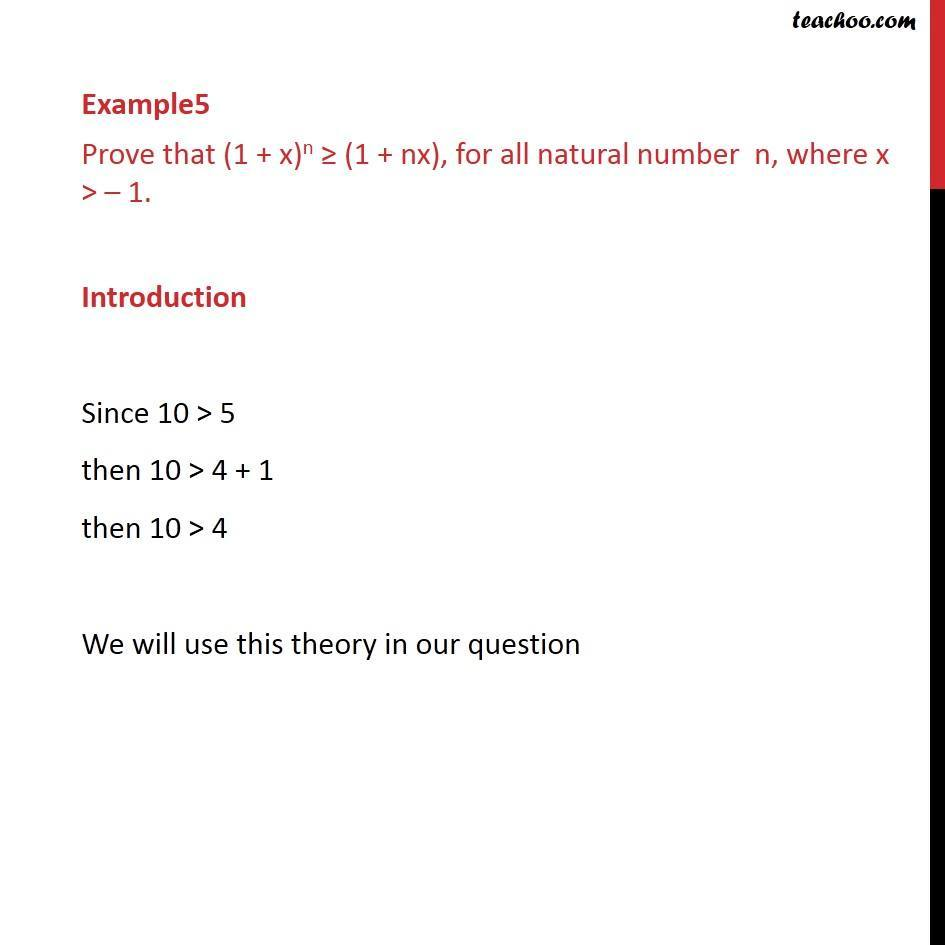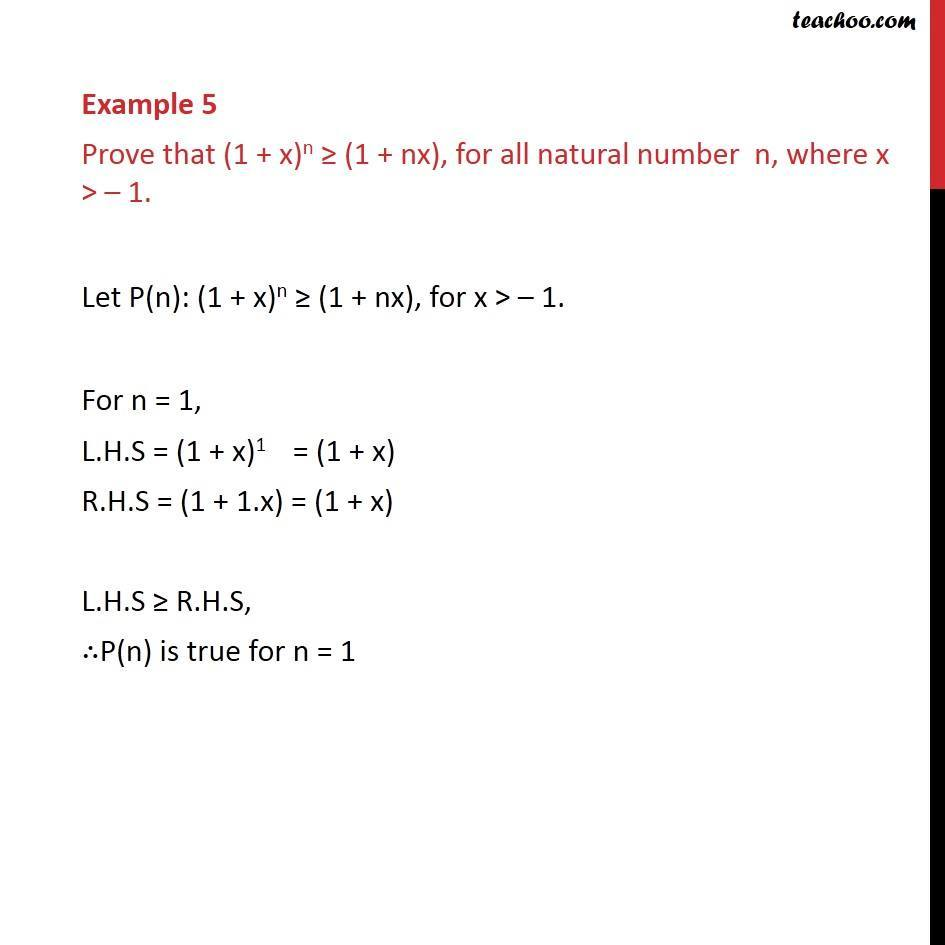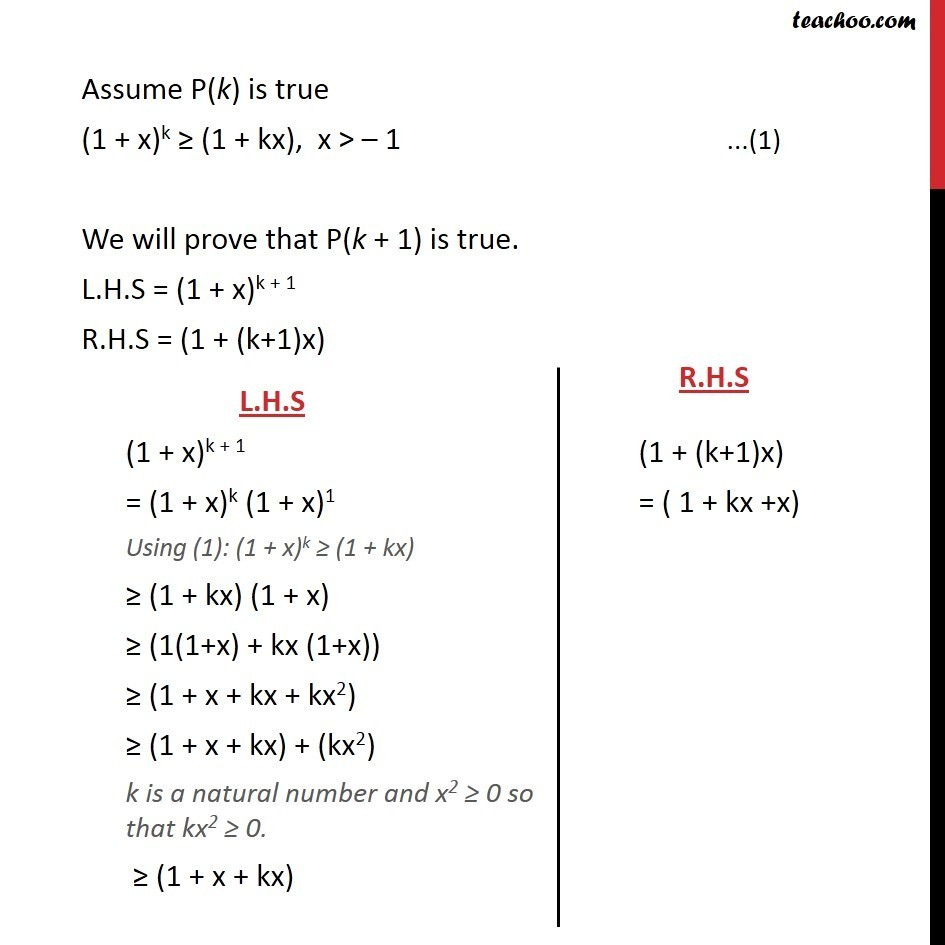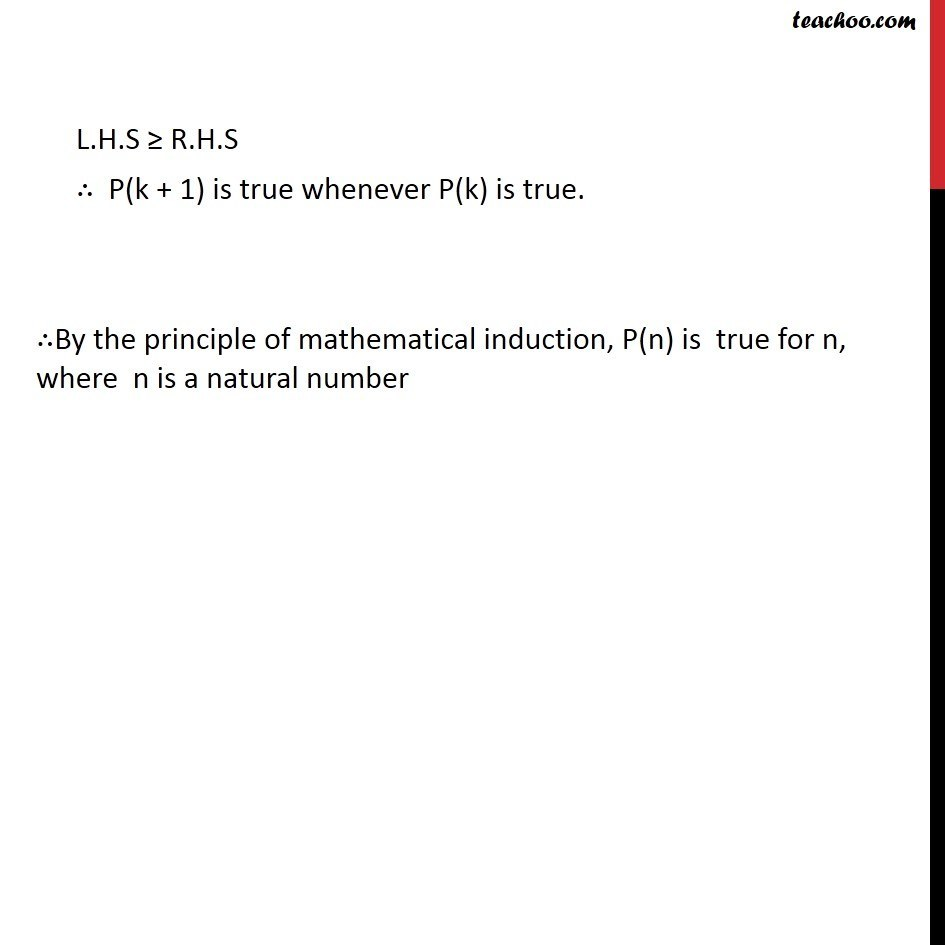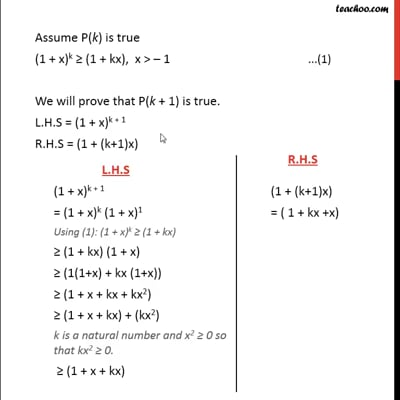This video is only available for Teachoo black users

### Transcript

Example5 Prove that (1 + x)n ≥ (1 + nx), for all natural number n, where x > – 1. Introduction Since 10 > 5 then 10 > 4 + 1 then 10 > 4 We will use this theory in our question Example 5 Prove that (1 + x)n ≥ (1 + nx), for all natural number n, where x > – 1. Let P(n): (1 + x)n ≥ (1 + nx), for x > – 1. For n = 1, L.H.S = (1 + x)1 = (1 + x) R.H.S = (1 + 1.x) = (1 + x) L.H.S ≥ R.H.S, ∴P(n) is true for n = 1 Assume P(k) is true (1 + x)k ≥ (1 + kx), x > – 1 We will prove that P(k + 1) is true. L.H.S = (1 + x)k + 1 R.H.S = (1 + (k+1)x) L.H.S ≥ R.H.S ∴ P(k + 1) is true whenever P(k) is true. ∴By the principle of mathematical induction, P(n) is true for n, where n is a natural number